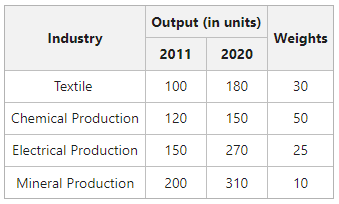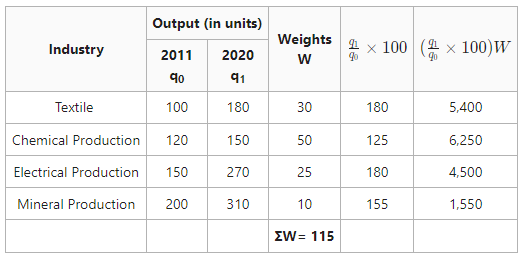# Index Number of Industrial Production: Meaning, Characteristics, Construction, and Example

The index number was first constructed by an Indian Statistician, Carli in 1764. It was used for the first time to compare the prices of the year 1750 with that of the year 1500.  An index number is a statistical tool used to measure changes in the magnitude of a group of related variables.

An index number is a statistical measure designed to show changes in variable or a group of related variables with respect to time, geographical location or other characteristic. – Spiegal

### What do you mean by Index Number of Industrial Production?

The index number of industrial production is prevailing these days.  The changes in the level of industrial production across many industries are measured by the index number of industrial production. Simply put, it measures an increase or decrease in a country’s industrial output relative to the base year.

#### Characteristics of Index Number of Industrial Production

1. The index number indicates changes in the rate of production.
2. The base year for the current series is 2011-2012 in India.
3. These index figures can be used to predict the growth of the economy’s industrial production.
4. The output of some industries may increase whereas others’ output might decline during a given period.
5. These are designed to evaluate changes in the level of industrial production across a given period with respect to a base period.
6. It evaluates changes in production volume rather than production value.

## Construction of Index Number of Industrial Production

Construction of the Index number of Industrial Production involves the following steps:

#### 1. Classification of Industries:

First of all, to the construct Index number of Industrial Production, the industries are categorized into:

(i) Mining Industries: Petroleum, coal, aluminium, iron ore, etc.

(ii) Metallurgical Industries: Rolling mills, iron, steel, etc.

(iii) Mechanical Industries: Aeroplanes, ships, locomotives, etc.

(iv) Industries related to the Excise Duties: Tabacco, sugar, match, etc.

(v) Textile Industries: Jute, silk, cotton, woollen, etc.

(vi) Miscellaneous: Soap, chemicals, glass, cement, etc.

#### 2. Collection of Statistics or Data Related to Industrial Production:

The next step includes the collection of data relating to the above-mentioned industries. The data can be collected either monthly, quarterly, or annually.

#### 3. Assigning Weightages:

Weights are assigned based on the relative importance of certain industries. The weights are determined by the value of respective industries’ net output and how much they contribute to the national income.

#### 4. Apply the Formula:

It involves the following steps:

• Consider the base year’s output (q0) to be 100 and express the production of the current year (q1) as a percentage of the base year’s production; i.e., (\frac{q_1}{q_0}\times100)
• Now multiply the calculated percentages by their relative weights and determine their total; i.e., calculate.
• Apply the formula of Index Number of Industrial Production:#### Example:

Construct the index number of industrial production from the following data:#### Solution:The Index Number of 153.91 shows that Industrial Production increased by 53.91% in the year 2020.